A couple of days ago, Peter Rowlett posted on The Aperiodical: Introducing hexboard – a LaTeX package for drawing games of Hex.

Hex is a strategic game with two players (Red and Blue) taking turns placing a stone of their color onto any empty space. A player wins when they successfully connect their sides together through a chain of adjacent stones.

Here’s a short game on a $5 \times 5$ board (normal play uses $11\times 11$ boards), won by Blue, drawn with the LaTeX-package hexboard.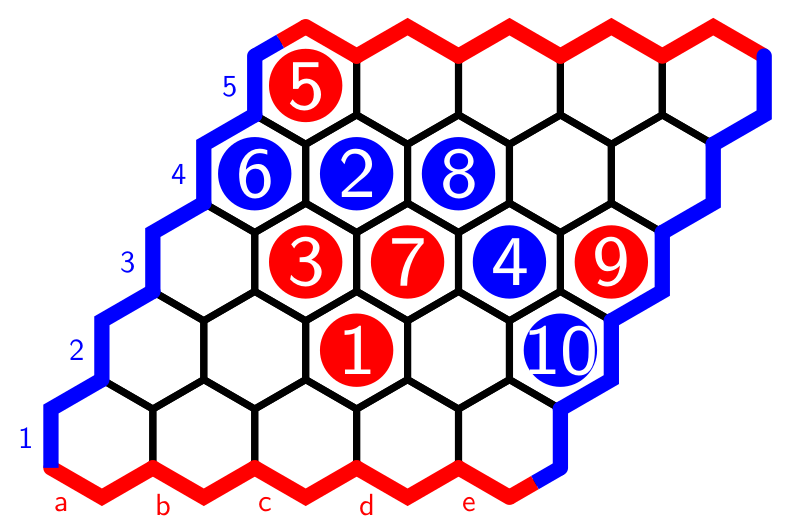As much as I like mathematical games, I want to use the versability of the hexboard-package for something entirely different: drawing finite Heyting algebras in which it is easy to visualise the logical operations.

Every full hexboard is a poset with minimal cell $0$ and maximal cell $1$ if cell-values increase if we move horizontally to the right or diagonally to the upper-right. With respect to this order, $p \vee q$ is the smallest cell bigger than both $p$ and $q$, and $p \wedge q$ is the largest cell smaller than $p$ and $q$.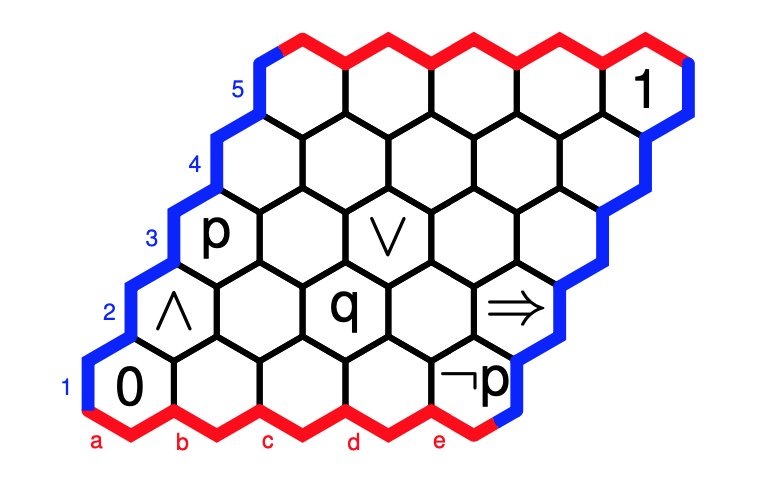The implication $p \Rightarrow q$ is the largest cell $r$ such that $r \wedge p \leq q$, and the negation $\neg p$ stands for $p \Rightarrow 0$. With these operations, the full hexboard becomes a Heyting algebra.

Now the fun part. Every filled area of the hexboard, bordered above and below by a string of strictly increasing cells from $0$ to $1$ is also a Heyting algebra, with the induced ordering, and with the logical operations defined similarly.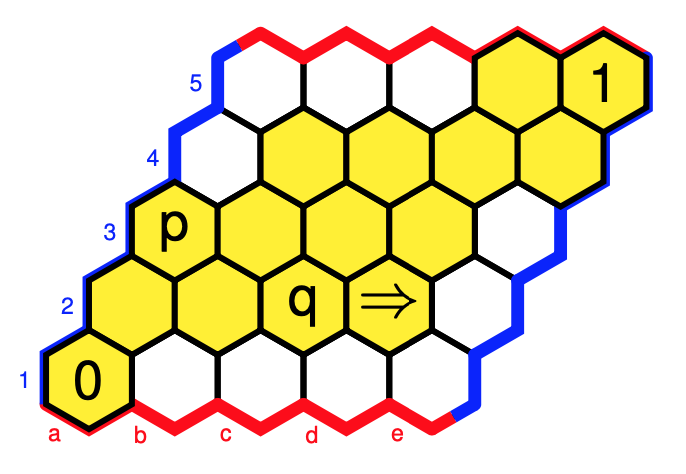Note that this mustn’t be a sub-Heyting algebra as the operations may differ. Here, we have a different value for $p \Rightarrow q$, and $\neg p$ is now $0$.

If you’re in for an innocent “Where is Wally?”-type puzzle: $W = (\neg \neg p \Rightarrow p)$.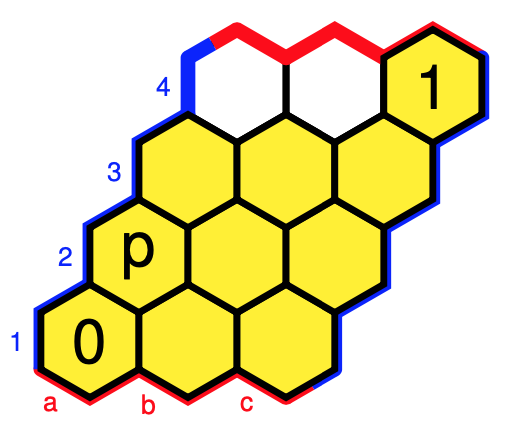Click on the image to get the solution.

The downsets in these posets can be viewed as the open sets of a finite topology, so these Heyting algebra structures come from the subobject classifier of a topos.

There are more interesting toposes with subobject classifier determined by such hex-Heyting algebras.

For example, the Topos of Triads of Thomas Noll in music theory has as its subobject classifier the hex-Heyting algebra (with cell-values as in the paper):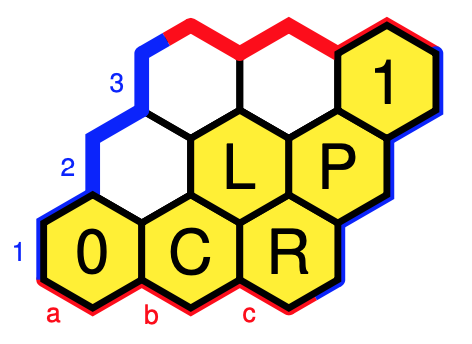Note to self: why not write a couple of posts on this topos?

Another example: the category of all directed graphs is the presheaf topos of the two object category ($V$ for vertices, and $E$ for edges) with (apart from the identities) just two morphisms $s,t : V \rightarrow E$ (for start- and end-vertex of a directed edge).

The subobject classifier $\Omega$ of this topos is determined by the two Heyting algebras $\Omega(E)$ and $\Omega(V)$ below.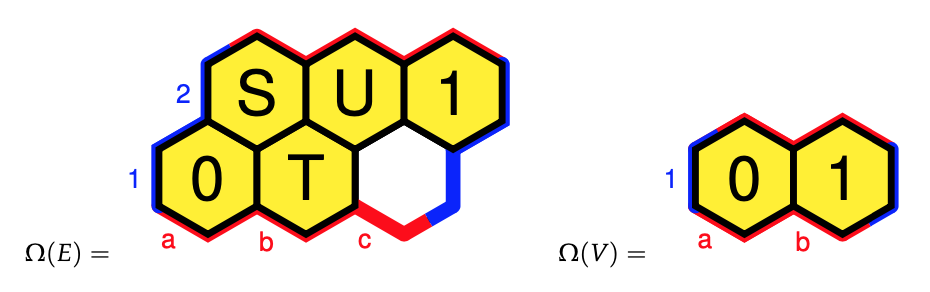These ‘hex-Heyting algebras’ are exactly what Eduardo Ochs calls ‘planar Heyting algebras’.

Eduardo has a very informative research page, containing slides and handouts of talks in which he tries to explain topos theory to “children” (using these planar Heyting algebras) including:

Perhaps now is a good time to revive my old sga4hipsters-project.

Published in books games geometry math